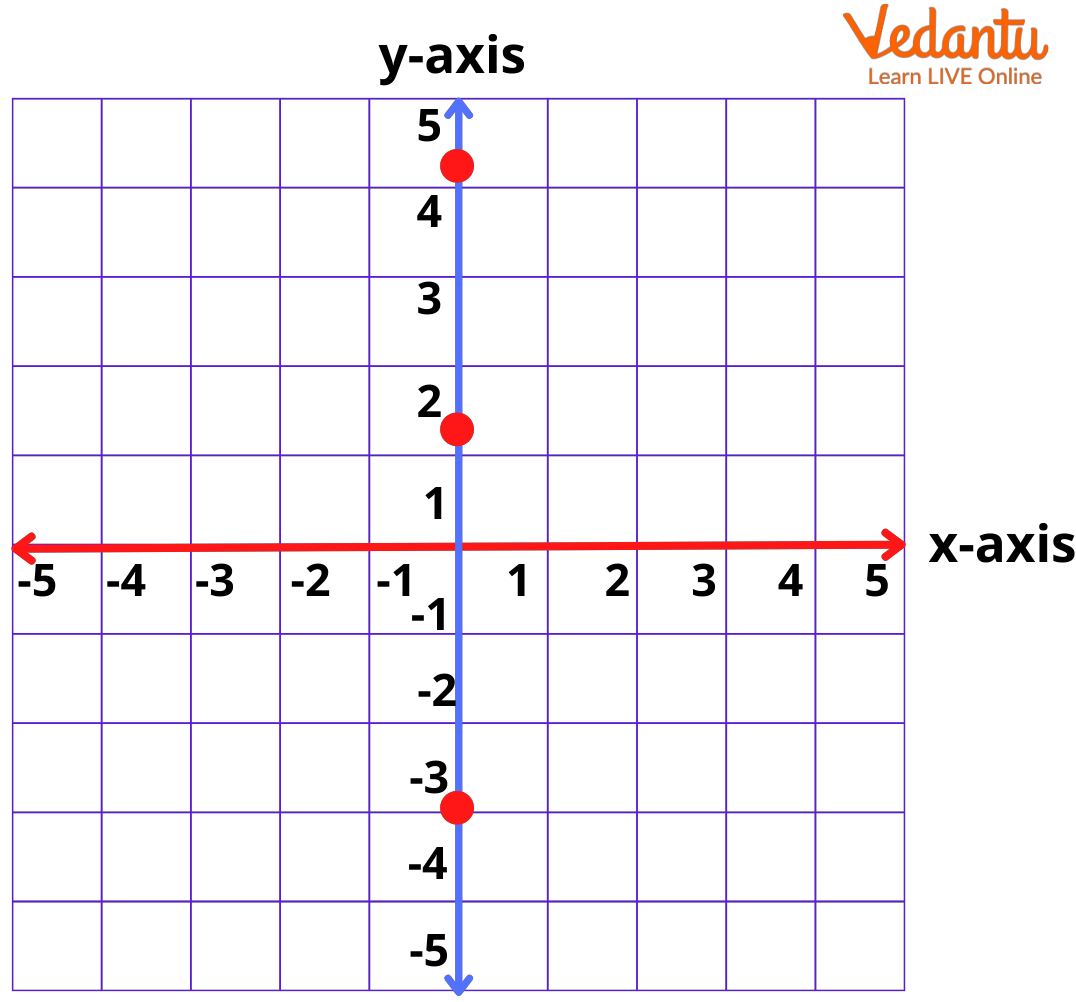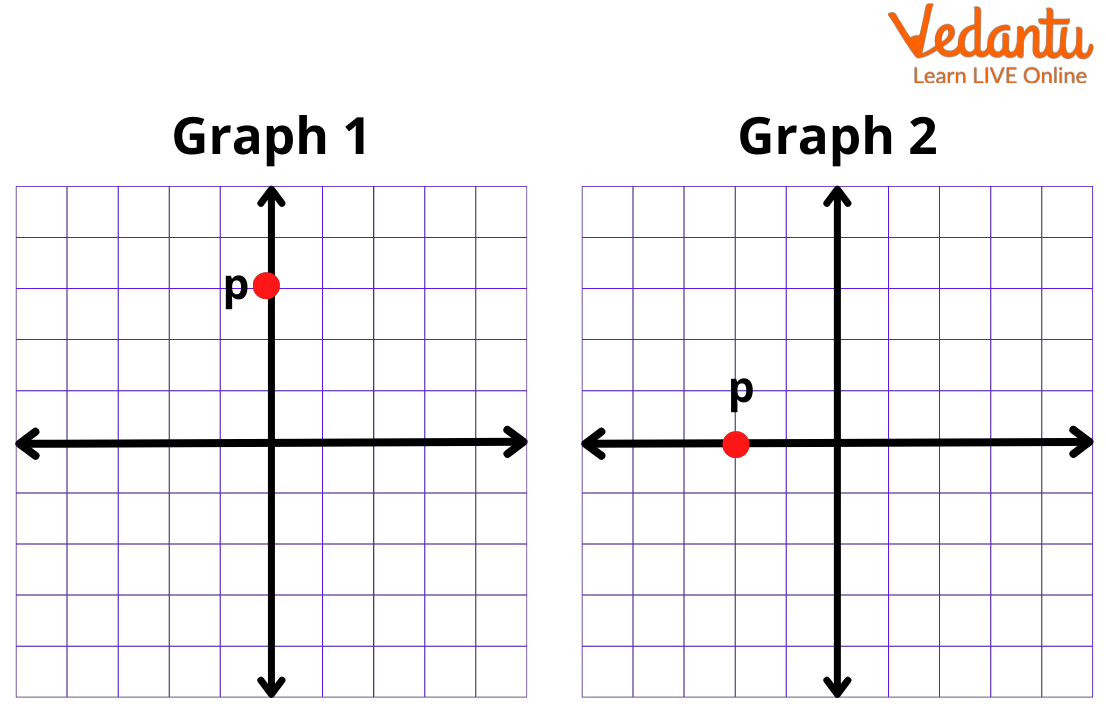Courses
Courses for Kids
Free study material
Free LIVE classes
More

# X Axis ExamplesLIVE
Join Vedantu’s FREE Mastercalss

## What is an X-Axis?

When you enter your room, you start moving across the floor and see one standing line or a beam too. So, what is this floor and a beam are? Well, the floor is an x-axis and the beam standing on the floor is a y-axis. In the reverse case, if you consider the beam as an x-axis, the floor becomes your y-axis, but what are these x and y-axis?

Well, if you look at the following image, you will clearly understand what an axis is:Here, on this page, we will understand all about an x-axis along with certain x axis  examples.

## What Do You Understand by Axis?

From the above text, we understand that the floor is horizontal and the beam is vertical (that is standing). Also, this horizontal line is an axis, that’s why we call the x-axis the horizontal axis as well.

Furthermore, the y-axis is across a vertical line, so we call it a vertical axis as well.

Now, let us see some questions to understand what an x-axis is.

## X Axis Examples

1. Which of the following graphs do you find P on the x-axis?• Graph 1

• Graph 2

• None of these

Ans: Option B

Here, we have two graphs: Graph 1 and Graph 2. Here, graph 1 has P lying along the vertical line/vertical axis or y-axis.

In Graph 2, we have P lying along the horizontal line or horizontal axis or an x-axis.

So, we confirm that graph 2 has P lying on an x-axis, which is our required answer.

2. Which of the following points lie on an x-axis?

• 1, 1

• 1, 0

• 0, 1

• −1, −1

Ans: Option b

Here, we need to understand that we are focusing on the x-axis only, which means that the value of a point on the y-axis must be eliminated and it must be zero.

So, let us see which coordinate has the y-axis as zero:

Please note that points that lie on a graph are coordinates. Here,

• 1, 1  means 1 is on the x-axis and 1 is one y-axis

• 1 lies on the x-axis and 0 on the y-axis

• 0 on the x-axis and 1 on the y-axis

• - 1 on the x-axis and -1 on the y-axis

We notice that option b has the y-axis as zero, which means that the point (1, 0) lies on the axis only (not considering the y-axis here).

Lastly, we understand the following differences between the x and the y-axis on a graph:

From the above text, we understand the following points:

• The coordinates of the middle of the origin of a graph ‘0’ are (0, 0).

• If a point lies on the x-axis, then the point on the y-axis is 0. So if there is a point A on the x-axis, its coordinates become (x, 0).

• However, if the point lies on the y-axis, then the point on the x-axis is zero. So if there is a point A on the y-axis, its coordinates become (0, y).

• The arrows on the x and the y-axis suggest that both lines are endless.

Last updated date: 25th Sep 2023
Total views: 141.3k
Views today: 1.41k

## FAQs on X Axis Examples

1. If a point lies on the x-axis then what will be its y coordinate?

We know coordinate or a point is a number that represents the position of a point across a line or arc. The origin point is (0,0). As per our understanding, we notice that a point lying on the x-axis will have 0 as its y coordinate and thus, the point would be (x, 0).

2. How do we describe the x-axis versus the y-axis?

The proper title of a graph is "x-axis variable vs. y-axis variable." For example, if you are comparing the number of pages required to make any drawing, you notice that the number of pages would be the independent, or x-axis variable and the drawing is dependent or the y-variable.